# Determine the half-life of a nuclide that loses 83.0% of its mass in 427 hours. 167...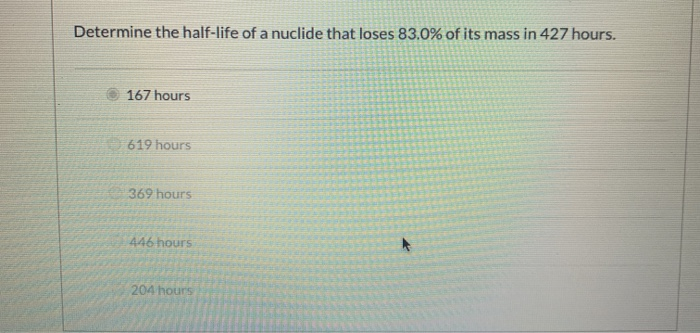Determine the half-life of a nuclide that loses 83.0% of its mass in 427 hours. 167 hours 619 hours 369 hours 446 hours 204 hours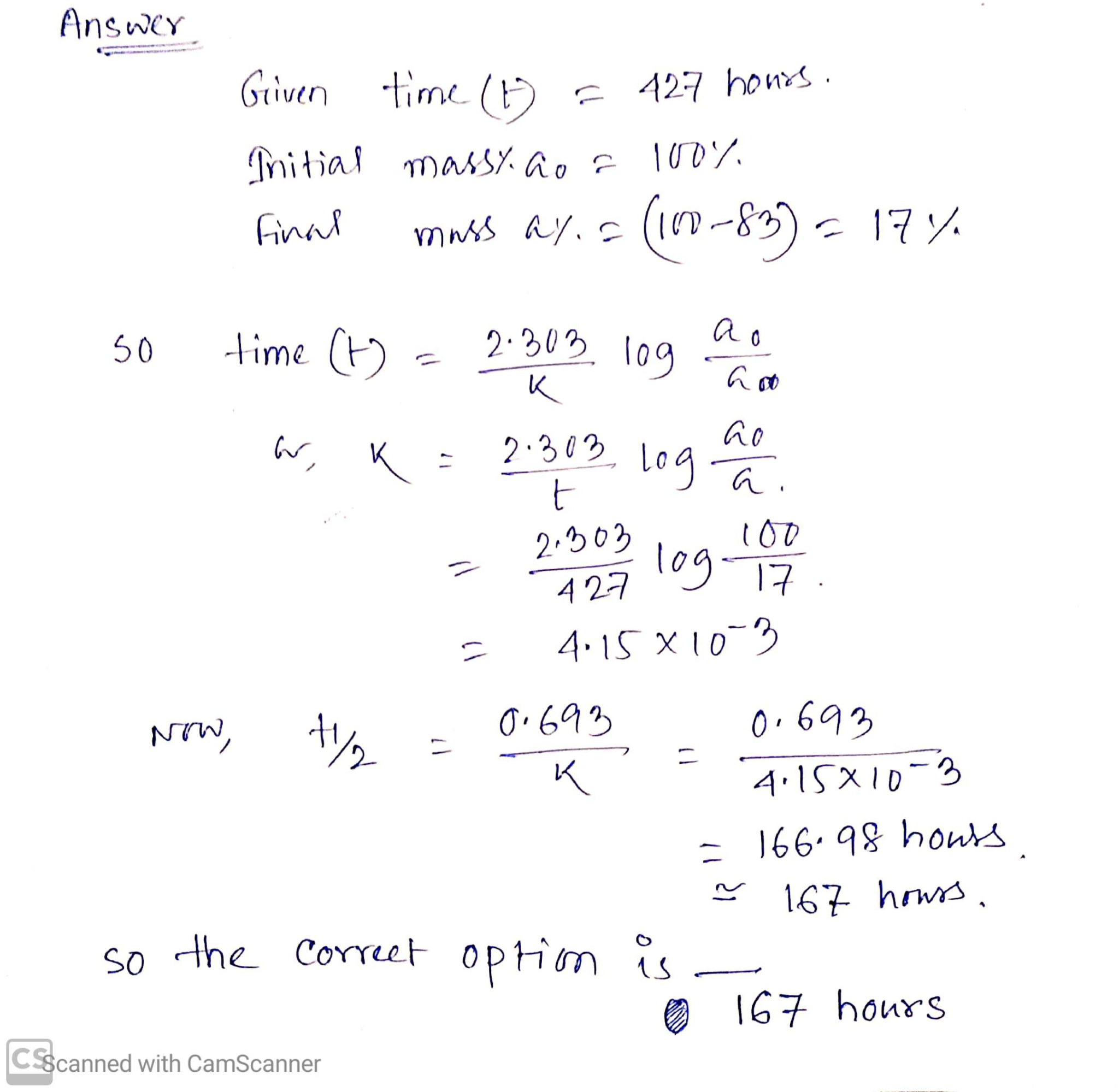##### Add Answer of: Determine the half-life of a nuclide that loses 83.0% of its mass in 427 hours. 167...
Similar Homework Help Questions
• ### Determine the half-life of a nuclide that loses 38.0% of its mass in 407 hours. (a),...

Determine the half-life of a nuclide that loses 38.0% of its mass in 407 hours. (a), 291 hours. (b), 568 hours. (c), 204 hours. (d), 586 hours.(e) 281 hours.

• ### 19. What will the mass of nuclide X be after 24 hours have elapsed? 20. What...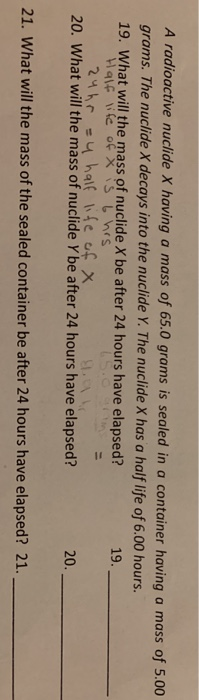19. What will the mass of nuclide X be after 24 hours have elapsed? 20. What will the mass of nuclide Y be after 24 hours have elapsed? 21. What will the mass of the sealed container be after 24 hours have elapsed? A radioactive nuclide X having a mass of 65.0 grams is sealed in a container having a mass of 5.00 grams. The nuclide X decays into the nuclide Y. The nuclide X has a half life of...

• ### A radioactive nuclide X having a mass of 65.0 grams is sealed in a container having...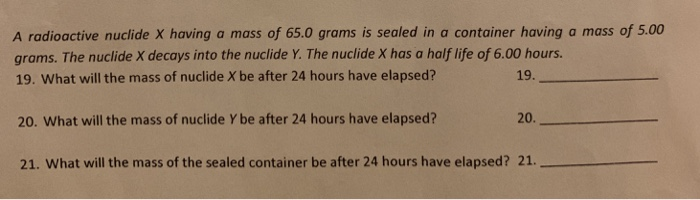A radioactive nuclide X having a mass of 65.0 grams is sealed in a container having a mass of. 5.00 grams. The nuclide X decays into the nuclide Y. The nuclide X has a half life of 6.00 hours. 19. What will the mass of nuclide Y be after 24 hours have elapsed? 20. What will the mass of nuclide Y be after 24 hours have elapsed? 21. What will the mass of the sealed container be after 24 hours...

• ### 8. The nuclide 76 As has a half-life of 26.0 hrs. If a sample of "As...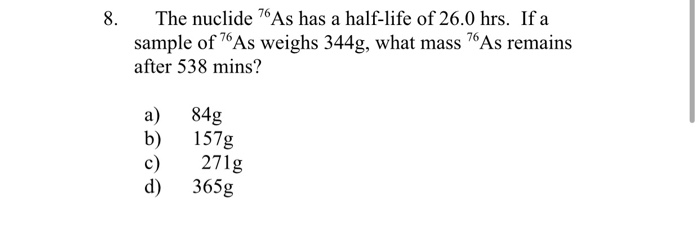8. The nuclide 76 As has a half-life of 26.0 hrs. If a sample of "As weighs 344g, what mass 7As remains after 538 mins? a) b) c) d) 84g 157g 271g 365g Show transcribed image text

• ### The Cs-131 nuclide has a half-life of 30 years. After 120 years, 8.7 g remain. What...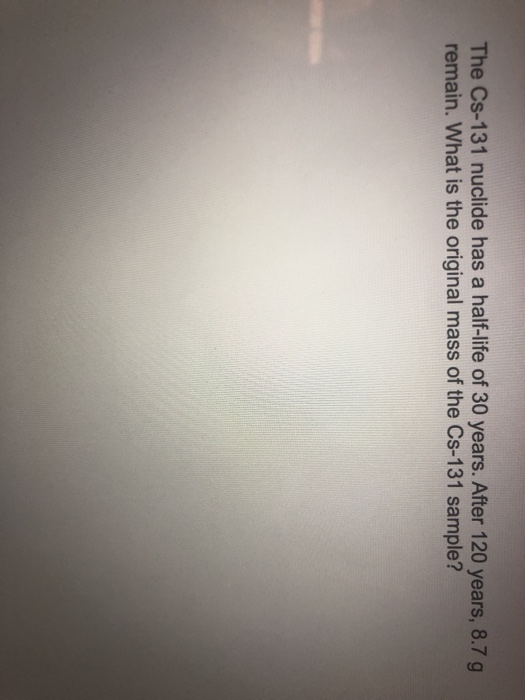The Cs-131 nuclide has a half-life of 30 years. After 120 years, 8.7 g remain. What is the original mass of the Cs-131 sample? Show transcribed image text

• ### Sodium-24 is used to study blood circulation and has a half-life of 15 hours. Which one...

Sodium-24 is used to study blood circulation and has a half-life of 15 hours. Which one of the following statements is true? a. It is not possible to know exactly how much remains after 45 hours because the rate of decay depends on the initial amount. b. After 45 hours have elapsed, all of the nuclide will have decayed. c. After 45 hours have elapsed, 1/8 of the original amount remains d. After 45 hours have elapsed, 1/4 of the...

• ### Sodium-24 is used to study blood circulation and has a half-life of 15 hours. Which one...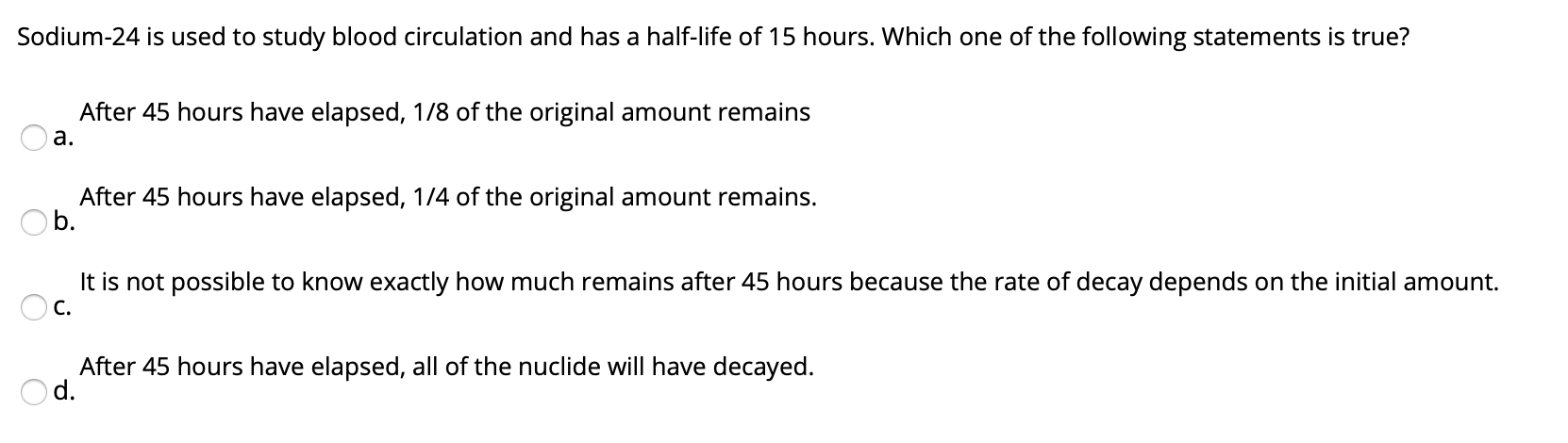Sodium-24 is used to study blood circulation and has a half-life of 15 hours. Which one of the following statements is true? After 45 hours have elapsed, 1/8 of the original amount remains a. After 45 hours have elapsed, 1/4 of the original amount remains. Ob. It is not possible to know exactly how much remains after 45 hours because the rate of decay depends on the initial amount. Oc. After 45 hours have elapsed, all of the nuclide will...

• ### step by step please! need help understanding 6. Terbium-152 is a positron emitter with a half-life of 18.0 hours. Ho...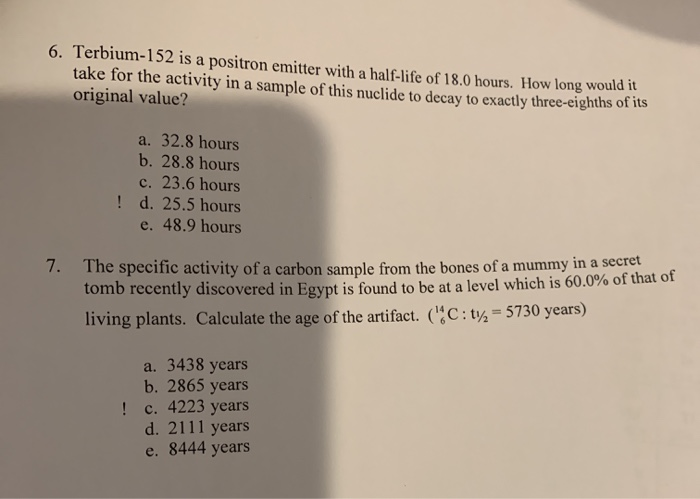step by step please! need help understanding 6. Terbium-152 is a positron emitter with a half-life of 18.0 hours. How long would take for the activity in a sample of this nuclide to decay to exactly three-eighths of its original value? a. 32.8 hours b. 28.8 hours c. 23.6 hours ! d. 25.5 hours e. 48.9 hours 7. The specific activity of a carbon sample from the bones of a mummy in a secret como recently discovered in Egypt is...

• ### a) Radium-230 has a half-life of 93 hours. Which one of the following statements is true?...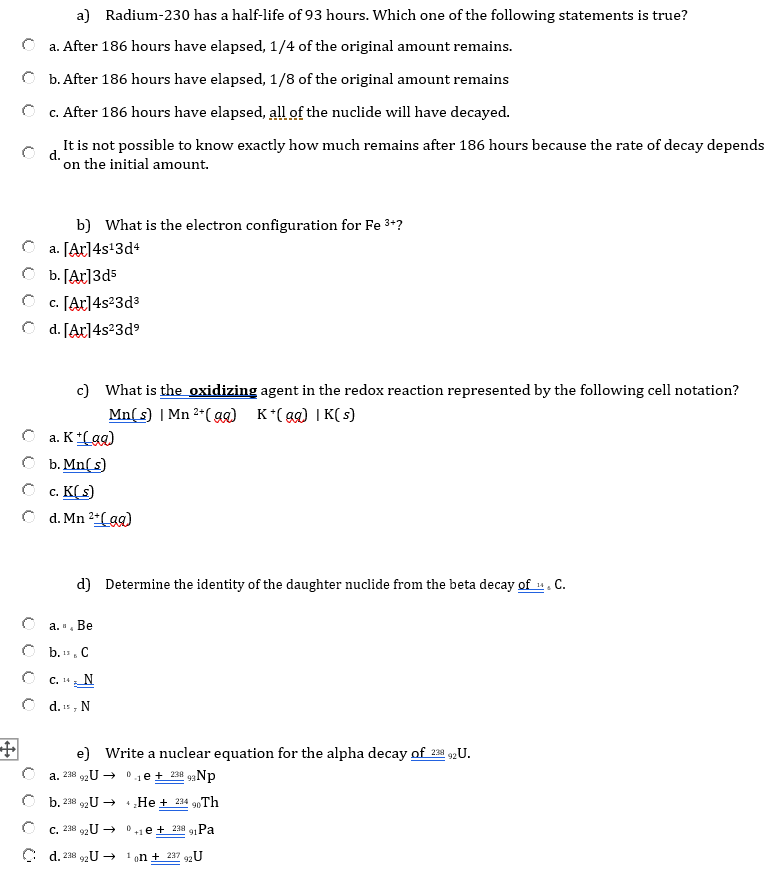a) Radium-230 has a half-life of 93 hours. Which one of the following statements is true? a. After 186 hours have elapsed, 1/4 of the original amount remains. O b. After 186 hours have elapsed, 1/8 of the original amount remains c. After 186 hours have elapsed, all of the nuclide will have decayed. It is not possible to know exactly how much remains after 186 hours because the rate of decay depends d. on the initial amount. b) What...

• ### An unknown radioactive substance has a half-life of 3.20 hours . If 14.8 grams of the substance...

Part B An unknown radioactive substance has a half-life of 3.20 hours . If 14.8 grams of the substance is currently present, what mass of Ao was present 8.00 hoursago?Part C Americium-241 is used in some smoke detectors. It is an alpha emitter with a half-life of 432 years . How long will it take in years for 43.0% of an Am-241sample to decay?

Free Homework App